You may also like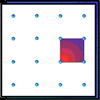Geoboards

This practical challenge invites you to investigate the different squares you can make on a square geoboard or pegboard.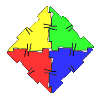Polydron

This activity investigates how you might make squares and pentominoes from Polydron.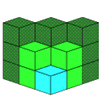If you had 36 cubes, what different cuboids could you make?

Combining Cuisenaire

Age 7 to 11 Challenge Level:

Here are two different Cuisenaire rods: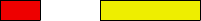How many different ways can you find to line them up end to end?
How many different ways are there to line them up if both rods are the same?

Now there are three different rods: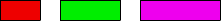In how many different ways can you line up the three rods?
Imagine two of the rods are the same. How many different ways are there to line them up now?
How many different ways are there if all the rods are the same?

What would happen if there were four different rods? How many ways can you line them up now?
If two rods are the same, how many ways could you line them up?
And how many ways are there to line them up if three are the same?
How about if they are all the same?

You might like to use the interactivity below to try out your ideas.
Full Screen Version